# 1 bookdown使用

bookdown是谢益辉之前开发的R语言包，可以基于rmarkdown快速生成在线电子书，并且可以输出pdf和epub。具体的使用方法可以参见官方文档。

https://bookdown.org/yihui/bookdown/

https://www.math.pku.edu.cn/teachers/lidf/docs/Rbook/html/_Rbook/

• Latex公式在在$与公式间不要空格。 • 插入图片建议使用r的function。例子如下： 其中include_graphics括号后面为图片路径。同时在R code的设置里，在设置图片大小时推荐使用out.width和out.height参数，设置为’100%’，这样图片可以根据排版情况进行自适应。 • 如果想加入r代码块而不想运行仅作为展示的话，需要在R code的设置里设置为r eval=FALSE, echo = T。 • 目前这个版本的封面图片设置参数cover-image只能生成epub里的封面，pdf无法添加。 • 默认模板会生成图和表目录，不需要的可以在index.rmd的输出设置里把lot和lof设置为false。 • 默认模板pdf里有一句话“献给……呃，爱谁谁吧”，需要在模板的latex文件夹下的before_body.tex里去掉。 • 默认模板设置是B5的纸张大小，边距设置也是左右不对称。这个是在index.rmd的输出设置里。实际上也是latex的设置。可根据自己喜好做调整。 • 用github托管的话，可以在bookdown.yml文件里设置输出文件夹参数，在最后一行添加参数（output_dir: “docs”）。然后在github的Pages设置对应的根目录。同时需要在R里输入如下命令。让网页不使用默认jekyll主题。 最后奉上最新的bookdown在线电子书地址： http://gisersqdai.top/Note-of-Applied-Statistics-with-R-Book/ # 2 公式更正 在修改的过程里，我发现了来自BruceZhaoR同学18年的一条pr，虽然不知道什么原因我一直没注意到这条pr。这里郑重向这位同学道歉，非常感谢他的指正。他指出在原本第三章描述性统计里的样本方差与标准差公式里有误。并给出了wiki上的参考公式。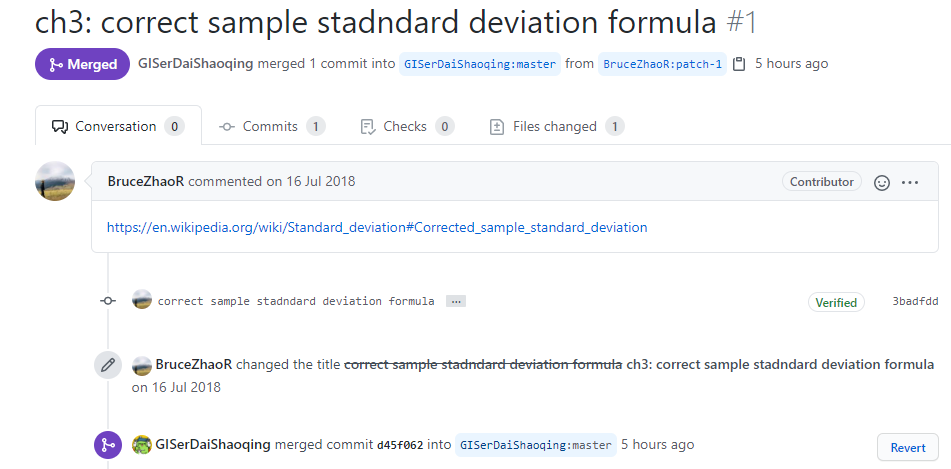具体错误这里也说明下。原公式如下： 样本方差： $$s^2=\frac{\sum_{i=1}^N (x_i-\mu)^2}{n-1}$$ $$s^2=\frac{\sum_{i=1}^k (M_i-\mu)^2f_i}{n-1}$$ 样本标准差： $$s=\sqrt {\frac{\sum_{i=1}^N (x_i-\mu)^2}{n-1}}$$ $$s=\sqrt{\frac{\sum_{i=1}^k (M_i-\mu)^2f_i}{n-1}}$$ 这里用$\mu$是不对的，$\mu$虽然可以指代统计学中的均值，但是$\mu$是代表总体均值。而严格来说，样本均值通常只是近似总体均值，因此必须作区分，故常用$\bar x$来做为样本均值。故修改后公式为 样本方差： $$s^2=\frac{\sum_{i=1}^N (x_i-\bar x)^2}{n-1}$$ $$s^2=\frac{\sum_{i=1}^k (M_i-\bar x)^2f_i}{n-1}$$ 样本标准差： $$s=\sqrt {\frac{\sum_{i=1}^N (x_i-\bar x)^2}{n-1}}$$ $$s=\sqrt{\frac{\sum_{i=1}^k (M_i-\bar x)^2f_i}{n-1}}$$ # 3 OR值计算 由于我目前主要从事健康地理学方面的研究，最近碰上了一个基础的OR值计算问题。首先OR值的全称是odds ratio值，这是公共卫生领域的一个专业名词。这里给出Encyclopedia of Public Health的定义。 The odds ratio (OR) provides a measure of the strength of relationship between two variables, most commonly an exposure and a dichotomous outcome. It is most commonly used in a case control study where it is defined as “the ratio of the odds of being exposed in the group with the outcome to the odds of being exposed in the group without the outcome.” This concept can be extended to a situation with multiple levels of exposure (e.g., low, moderate, or high exposure to an environmental containment). One exposure level is assigned as the “reference” level. For each of the remaining exposure levels, one divides the odds of that exposure level in the outcome positive group (compared with the reference level) by the odds of that exposure level in the outcome negative group. The OR ranges in value from 0 to infinity. Values close to 1.0 indicate no relationship between the exposure and the outcome. Values less than 1.0 suggest a protective effect, while values greater than 1.0 suggest a causative or adverse effect of exposure. 这里简单翻译一下，OR值是用来度量两个变量之间关系强度的指标，常见于暴露水平与二分的健康结局变量。最常用在案例对照研究。OR被定义为“组中暴露患病几率与暴露未患病几率的比值”。这个概念通常可以拓展到多水平暴露指标，通常定义某一类别的暴露水平为参考水平，对于剩余的暴露水平，则除以该参考水平的暴露几率用来进行比较。这里要先给出odds的定义。odds，称为几率、比值、比数，是指某事件发生的可能性(概率)与不发生的可能性（概率）之比。用p表示事件发生的概率，则：odds = p/(1-p)。OR值的公共卫生意义如下：范围从0到无穷大。接近1.0的值表示暴露与结果之间没有关系。小于1.0的值表示保护作用，而大于1.0的值表示暴露的致病性或不利影响。 针对一个标准的2x2流病表格（如下）。实际上OR值计算如下： $$暴露时患病几率=\frac{暴露时患病病例数}{未暴露时患病病例数}=\frac{a}{c}$$ $$暴露时未患病几率=\frac{暴露时未患病病例数}{未暴露时未患病病例数}=\frac{b}{d}$$ $$OR = \frac{a/c}{b/d}=\frac{ad}{bc}$$ Outcome +ve Outcome -ve Exposed a b No exposed c d 这里选用一个ICU的数据来进行说明，这个数据来源于David W. Hosmer等人出版的applied logistic regression一书中的数据。获取途径可以通过安装这个书的r包，命令如下。 安装完成以后，载入数据做个初步探索。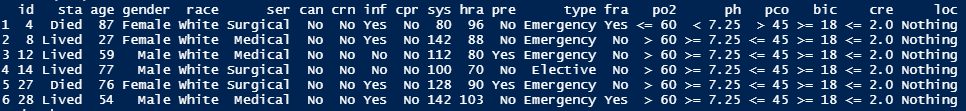为了简单化，我们这里定义健康结局变量为status，即数据中的sta（Lived或者Died），感兴趣的自变量为age。首先绘制一下图。由于status是个二分变量。所以图就变成了如下的样子。你是否觉得很熟悉？其实这就是logistic regression的典型数据。那么根据age的数据，我们做一个处理，统计不同年龄段的死亡率，以10岁为分界线。我们可以得到如下的图。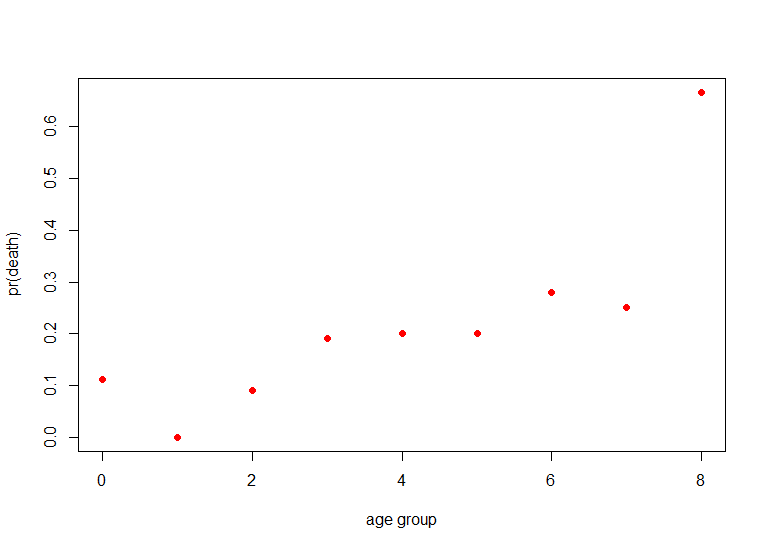那么我们突然发现，这个散点是有线性趋势的。假设我们采用线性回归来做分析，即假定有：$pr(death)=\beta_0+\beta_1(age)$，不就可以拟合了吗？但是我们又会发现一个问题。那就是这里的y(pr(death))是有现实意义的实数，也就是它的值域必须在（0,1）中。然而等式右边实际上是可以取任何值的（根据$\beta_0 , \beta_1, age$），因此这个线性方程即使求解出来，预测值通常会超过实际的值域。所以为了解决这个问题，logistics regression就提出了。首先是定义了logit函数为： $$logit(p)=log(\frac{p}{1-p})$$ $$p=pr(death)$$ 那么这个logit函数的现实意义是事件发生几率的对数。那么同时模型就变成了： $$log(\frac{p}{1-p})=\beta_0+\beta_1(age)$$ 这时我们就会发现p的值域是在（0,1），而logit(p)的值域则是$[ - \infty, + \infty ]$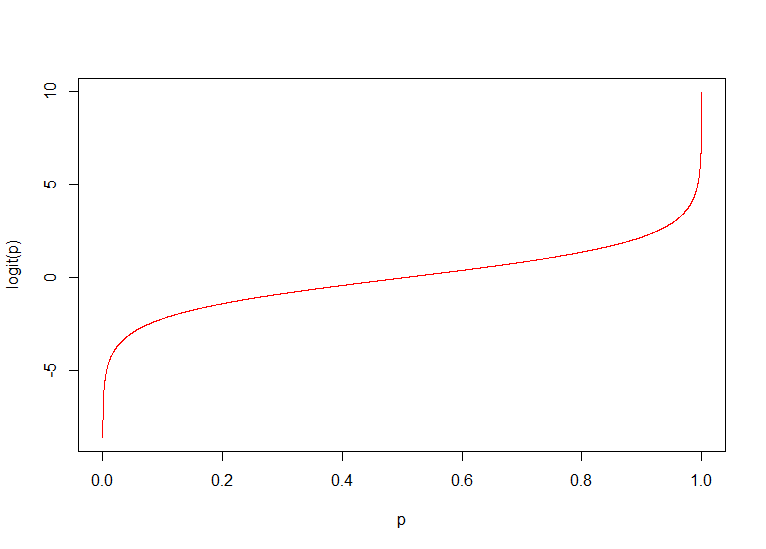那这个时候我们就可以用线性回归方法求解系数了，因此logistic regression也被称为广义线性回归的一类。 那么我们再来看一个更特殊的情况，就是前面说的2x2联表的情况。假定自变量是个分类变量。这里选用icu数据里的type来分析（健康结局变量不变）。也就是说方程如下： $$logit(p)=\beta_0+\beta1(I{type})$$ 2x2联表则为： Lived Died elective admission a b emeregency admission c d 那么这时候$I{type}=0$时是elective admission，$I{type}=1$时是emeregency admission。因此我们可以得到对应的y值。也就是elective adminssion的logit(p)为$\beta_0$。而emergency admission的logit(p)为$\beta_0+\beta_1\$。那么根据logit函数的定义，我们就有如下的式子：

$$odds_{ele}=\frac{p}{1-p}=\frac{a}{b}=e^{\beta_0}$$

$$odds_{eme}=\frac{p}{1-p}=\frac{c}{d}=e^{\beta_0+\beta_1}$$

$$OR =\frac{ad}{bc} =odds{ele}/odds{eme}=\frac{a}{b}/\frac{c}{d}=e^{\beta_0+\beta_1}/e^{\beta_0}=e^{\beta_0+\beta_1-\beta_0}=e^{\beta_1}$$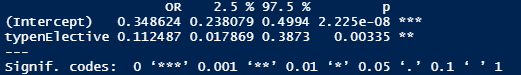Note-of-Applied-Statistics-with-R

Note-of-Applied-Statistics-with-R-Book